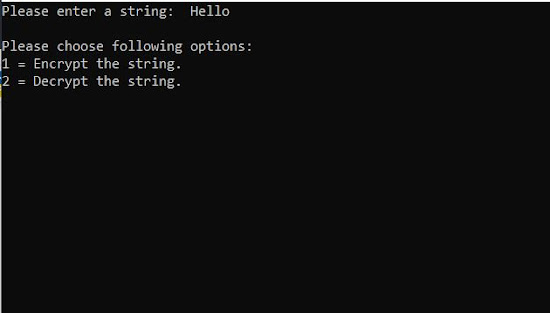# Encryption and Decryption project in C++ | C++ program for encryption and decryption of file

## Introduction

Hello, learners. Today we will learn a new concept and then create a project using C++ in that concept. Our topic for today is the Simple Encryption and Decryption project in C++;
But, before going into the project, we will see what encryption and decryption are and how to do it?
Although we won't build a super-strong encryption algorithm, we will see an easy one as a project.

## What is Cryptography?

Before we talk about our topic, let's see what cryptography means. Cryptography is the study of a secure way of transmitting data from person to person, where the data is modified only to be understandable by the intended persons.
In short, if we want to send data or messages securely, then we use cryptographic techniques to avoid the intervention of unwanted persons or systems.

## Encryption and Decryption

So, where do these terms come in the process of cryptography?
Encryption is a process that transforms the original information into an unrecognizable form.  The format of the secure message can be either changing the position of the characters or applying an algorithm to completely change the words.
Decryption is reverse encryption, which transforms an encoded message into a human-readable language.

Both the processes use a key to encode or decode the text. The concept is as follows.
The sender has a key that they use to encode and the receiver has a key to decode. The difference is that the keys can be different or the same depending on the technique used.
If the key is the same for encryption and decryption, we call it the Public Key Algorithm. If the key for encoding and decoding is different, it is called the Private key Algorithm.
Let us see the project we will be making.

## User Interface and functions

In this project, we are using the same key i.e a public key algorithm. Let us see how to interface looks.
So, as we can see in the image, it is a straightforward project. We take the input from the user and ask them about the operation they want to choose.

##Functioning

How will it work? So, we are taking a fixed non-negative integer and then adding or subtracting from the character of a word. We Add the integer in encryption and Subtract it for decryption.
This technique is also known as the Caesar cipher technique. Although it is an old technique, this method can give an idea of how cryptography works.
Let's see the code for the project, followed by its explanation.

## Code

``````1:  #include <iostream>
2:  using namespace std;
3:
4:  int main()
5:  {
6:    int i, x;
7:    char str;
8:
9:    cout << "Please enter a string:\t";
10:    cin >> str;
11:
12:    cout << "\nPlease choose following options:\n";
13:    cout << "1 = Encrypt the string.\n";
14:    cout << "2 = Decrypt the string.\n";
15:    cin >> x;
16:
17:    //using switch case statements
18:    switch(x)
19:    {
20:     //first case for encrypting a string
21:     case 1:
22:       for(i = 0; (i < 100 && str[i] != '\0'); i++)
23:        str[i] = str[i] + 2; //the key for encryption is 3 that is added to ASCII value
24:
25:       cout << "\nEncrypted string: " << str << endl;
26:       break;
27:
28:     //second case for decrypting a string
29:     case 2:
30:       for(i = 0; (i < 100 && str[i] != '\0'); i++)
31:       str[i] = str[i] - 2; //the key for encryption is 3 that is subtracted to ASCII value
32:
33:     cout << "\nDecrypted string: " << str << endl;
34:     break;
35:
36:     default:
37:       cout << "\nInvalid Input !!!\n";
38:    }
39:    return 0;
40:  }
``````

## Code Explanation

In the main function, we are using a switch-case to choose between encryption or decryption.
In encryption, we are adding an integer to the character so that it changed to the character of the new ASCII value.

When it comes to decryption, we take the same encrypted text and then change it by subtracting the same integer.

## Conclusion

So, in this article, we learned the concept of cryptography and created an encryption and decryption project using the caesar cipher. It is considered a beginner project to understand the string changing concept.

If you enjoyed this article, let us know in the comments and check out more C++ projects on our website.

Do check out more of our articles on web development as well

Happy Learning :)

Thank you.